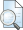# Analisa Komparasi Teknik Kompresi Citra Medis Menggunakan Run Length Encoding (RLE), Discrete Wavelet Transform (DWT) Dan Discrete Cosine Transform (DCT)

Mahmudi, Adnan and , Diah Priyawati, S.T, M.Eng (2017) Analisa Komparasi Teknik Kompresi Citra Medis Menggunakan Run Length Encoding (RLE), Discrete Wavelet Transform (DWT) Dan Discrete Cosine Transform (DCT). Skripsi thesis, Universitas Muhammadiyah Surakarta.PDF (Surat Pernyataan Publikasi) Surat Pernyataan adnan.pdf Download (271kB)PDF (Naskah Publikasi) TUGAS AKHIR adnan mahmudi L200130059.pdf Download (2MB)

## Abstract

Medical image compression is the process minimizes the image by way of removing the data information is not considered important in order to facilitate the hospital to manage data. Medical image compression is needed, in a study showing that a hospital can produce up to 5-15 GB of data per day. Research on medical image compression comparisons are indispensable in order to obtain the appropriate method of medical image compression that is needed at this time. This research uses three methods will be compared i.e. RLE, DWT and DCT. RLE method is the value of the degree classification faqs gray will then be encoded with the number of bits a little more. DWT method is a method that can provide information and data, thus making the scale becomes more accurate. DCT compression method is a method of compression that can change the signal into the frequency so that the image compression results obtained will simplify the delivery process. Calculation of value of PSNR, MSE, ratio and time as data validation and the comparison parameter. PSNR is the highest value value comparison of the magnitude of the noise while the value of the MSE is the value of the quadratic error between the original image with the image compression results. The ratio is a value that indicates the size of the compression results and shows what percentage of the image can be compressed while the time shows the efficiency of compression algorithms are used. Test data used in this research is medical image gets digitized using the resolution 200dpi and the secondary image. Application of MATLAB 2016a used to simplify the process of research and as documentation. Research on the comparison of medical image compression using DCT and DWT, RLE produce that the appropriate method is a method of RLE. RLE method have average PSNR values highest i.e. 35.67 dB, i.e. the lowest MSE 20.81 dB and fastest compression time i.e. 0.77 s while the method of DCT has the highest ratio of value i.e. 80.09% but the lowest of 28.66 PSNR value dB and the highest MSE of 91.85 dB, so that the appropriate method for medical image compression is currently the method of RLE.

Item Type: Karya ilmiah (Skripsi) medical image compression, RLE, DWT, DCT Q Science > QM Human anatomyT Technology > TA Engineering (General). Civil engineering (General) Fakultas Ilmu Komunikasi dan Informatika > Teknik Informatika ADNAN MAHMUDI 08 Nov 2017 07:43 08 Nov 2017 07:43 http://eprints.ums.ac.id/id/eprint/57041

### Available Versions of this Item

• Analisa Komparasi Teknik Kompresi Citra Medis Menggunakan Run Length Encoding (RLE), Discrete Wavelet Transform (DWT) Dan Discrete Cosine Transform (DCT). (deposited 08 Nov 2017 07:43) [Currently Displayed]View Item